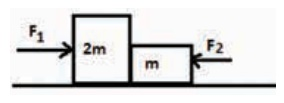Home | | Physics 11th std | Choose the correct answers

## Chapter: 11th Physics : UNIT 3 : Laws of Motion

Physics : Laws of Motion : Book Back Choose the correct Answers with Solution, One Mark and Multiple choice questions with Answers and Solution

Laws of motion | Physics

1. When a car takes a sudden left turn in the curved road, passengers are pushed towards the right due to

(a) inertia of direction

(b) inertia of motion

(c) inertia of rest

(d) absence of inertia

Answer a) : interia of direction

2. An object of mass m held against a vertical wall by applying horizontal force F as shown in the figure. The minimum value of the force F is(a) Less than mg

(b) Equal to mg

(c) Greater than mg

(d) Cannot determine

Solution:

f = mg

F = N

But f = μN = μF

F = f/μ = mg/μ

3. A vehicle is moving along the positive x direction, if sudden brake is applied, then

(a) frictional force acting on the vehicle is along negative x direction

(b) frictional force acting on the vehicle is along positive x direction

(c) no frictional force acts on the vehicle

(d) frictional force acts in downward direction

Answer: a) Frictional force acting on the vehicle is along negative direction

4. A book is at rest on the table which exerts a normal force on the book. If this force is considered as reaction force, what is the action force according to Newton’s third law?

(a) Gravitational force exerted by Earth on the book

(b) Gravitational force exerted by the book on Earth

(c) Normal force exerted by the book on the table

(d) None of the above

Answer: c) Normal force exerted by the book on the table

5. Two masses m1 and m2 are experiencing the same force where m1< m2. The ratio of their acceleration a1/a2 is

(a) 1

(b) less than 1

(c) greater than 1

(d) all the three cases

Solution:

F1 = F2

m1a1 = m2a2

a1 / a2 = m2 / m1

6. Choose appropriate free body diagram for the particle experiencing net acceleration along negative y direction. (Each arrow mark represents the force acting on the system).Solution:

Since the body is accelerating along y direction, the x component of force are unequal and more in negative y direction.

7. A particle of mass m sliding on the smooth double inclined plane (shown in figure) will experience(a) greater acceleration along the path AB

(b) greater acceleration along the path AC

(c) same acceleration in both the paths

(d) no acceleration in both the paths.

Answer: b) grater acceleration along the path AC

Solution:

The Force on the sliding object is mg sinθ As path AC is more slope than AB, greater accelaration along the path AC

8. Two blocks of masses m and 2m are placed on a smooth horizontal surface as shown. In the first case only a force F1 is applied from the left. Later only a force F2 is applied from the right. If the force acting at the interface of the two blocks in the two cases is same, then F1:F2: is(a) 1:1

(b) 1:2

(c) 2:1

(d) 1:3

Solution:

F1 = m1a = 2ma

F2 = m2a = ma

FI : F2

2ma : ma

2:1

9. Force acting on the particle moving with constant speed is

(a) always zero

(b) need not be zero

(c) always non zero

(d) cannot be concluded

Answer: b) need not be zero

Solution:

As in the case of particle moving in a circular path with constant speed has radial acceleration and hence centripetal force is acting.

10. An object of mass m begins to move on the plane inclined at an angle θ. The coefficient of static friction of inclined surface is μs. The maximum static friction experienced by the mass is

(a) mg

(b) μs mg

(c) μs mg sinθ

(d) μs mg cos θ

Solution:

For maximum static

Fsmax = μsN = μsmg cosθ

11. When the object is moving at constant velocity on the rough surface,

(a) net force on the object is zero

(b) no force acts on the object

(c) only external force acts on the object

(d) only kinetic friction acts on the object

Answer: a) net force on the object is zero

Solution:

Fext = − FFriction

Fnet = Fext + Ffri

= − Ffri + Ffri

Fnet = 0

12. When an object is at rest on the inclined rough surface,

(a) static and kinetic frictions acting on the object is zero

(b) static friction is zero but kinetic friction is not zero

(c) static friction is not zero and kinetic friction is zero

(d) static and kinetic frictions are not zero

Answer: c) static friction is not zero and kinetic friction is zero.

Solution:

As long as the body is at rest, the frictional force present between the contact surface is static friction only, when the object moves, the static friction disappears and kinetic friction come into play.

13. The centrifugal force appears to exist

(a) only in inertial frames

(b) only in rotating frames

(c) in any accelerated frame

(d) both in inertial and non-inertial frames

Answer: b) Only in rotating frames

14. Choose the correct statement from the following

(a) Centrifugal and centripetal forces are action reaction pairs

(b) Centripetal forces is a natural force

(c) Centrifugal force arises from gravitational force

(d) Centripetal force acts towards the center and centrifugal force appears to act away from the center in a circular motion

d) Centripetal force acts towards the centre and centrifugal force appears to act away from the center in circular motion

15. If a person moving from pole to equator, the centrifugal force acting on him

(a) increases

(b) decreases

(c) remains the same

(d) increases and then decreases

Solution:

Fcp = mw2R cos 90° = 0

Fce = mw2R cos 0° = mw2R

Fce > Fcp

pole θ = 90°, equator θ = 0°